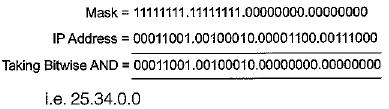Courses

# Introduction And IP Addressing (Advance Level) - 1

## 15 Questions MCQ Test GATE Computer Science Engineering(CSE) 2022 Mock Test Series | Introduction And IP Addressing (Advance Level) - 1

Description
This mock test of Introduction And IP Addressing (Advance Level) - 1 for Computer Science Engineering (CSE) helps you for every Computer Science Engineering (CSE) entrance exam. This contains 15 Multiple Choice Questions for Computer Science Engineering (CSE) Introduction And IP Addressing (Advance Level) - 1 (mcq) to study with solutions a complete question bank. The solved questions answers in this Introduction And IP Addressing (Advance Level) - 1 quiz give you a good mix of easy questions and tough questions. Computer Science Engineering (CSE) students definitely take this Introduction And IP Addressing (Advance Level) - 1 exercise for a better result in the exam. You can find other Introduction And IP Addressing (Advance Level) - 1 extra questions, long questions & short questions for Computer Science Engineering (CSE) on EduRev as well by searching above.
QUESTION: 1

### To prevent signal alternation, what is the max number of repeaters that can be placed on one 10 Base 5 or 10.Base 2 network?

Solution:

Maximum 4 repeaters can be placed in one transmission path between two nodes while 5 between two nodes.

QUESTION: 2

### Which IP class provides the fewest numbers of hosts?

Solution:

Number of host in class A = 224 - 2 Number of host in class B = 216 - 2 Number of host in class C = 28 - 2 Number of host in class D = 0

QUESTION: 3

### A repeater takes a weak and or corrupted signal and _____ it.

Solution:

A repeater takes a weak or corrupted signal and regenerate it

QUESTION: 4

A subnet mask in class A can have ______ 1’s with the remaining bits 0’s.

Solution:

Default subnet mask of class A network 11111111-00000000-00000000-00000000
⇒ 255-0-0-0.

QUESTION: 5

To interconnect two IP classes, class A and class C networks

Solution:

Router is used to connect two different class networks.

QUESTION: 6

Given an IP address 156.233.42.56 with a subnet mask of 7 bits. How many hosts and subnets are possible.

Solution: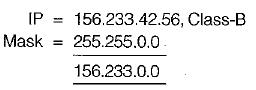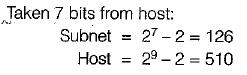QUESTION: 7

Class_______has the greatest number of hosts per given network address.

Solution:

Number of hosts in class A = 224 - 2 Number of hosts in class B = 216 - 2 Number of hosts in class C = 28 - 2

QUESTION: 8

The effective bandwidth is based on ______ .

Solution:

The effective data burst is a measure of average data rate, peak data rate and maximum data rate.

QUESTION: 9

Identify the unequal pair(s):

Solution:

Physical address is also known as MAC address. Logical address is also known as IP address COM1 is a communication port.

QUESTION: 10

There are three IP addresses as given below:
X = 202.23.14.150
Y = 168.19.200.12
Z = 72.192.52.210

Which of the following statements is/are correct?

Solution:

In IP add re sse s class A, B, and C used for general purpose.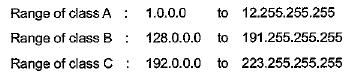QUESTION: 11

The default subnet mask for a Class B network can be

Solution:

If IP address is AND with subnet mask result will network address.
Default subnet mask:
Class A = 255.0.0.0
Class B = 255.255.0.0
Class C = 255.255.255.0

QUESTION: 12

Match List-I (Function) with List-11 (Layer) and select the correct answer using the codes given below the lists: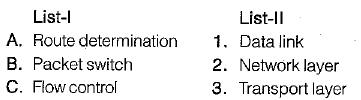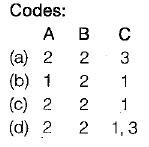Solution:

Packet switching→ network iayer.
Route determination→ 4 network layer.
Flow control → datalink and transport layer.

QUESTION: 13

Match List-I (Function) with List-lI (Layer) and select the correct answer using the codes given below the lists

List-I
A. Reassembly of packets
B. Responsibility for delivery between adjacent nodes
C. Mechanical electrical and functional interface
D. Error correction and retransmission

List-II
1. Transport
2. Data link
3. Physical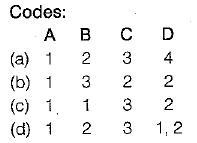Solution:
• Reassembly of packets is done at transport iayer.
• Responsibility for delivery between adjacent nodes is of data link layer.
• Mechanical electrical and functional interface is related to physical layer.
•  Error correction and retransmission is done at data link layer as well as transport layer.
QUESTION: 14

A subnet mask in class A has fourteen 1’s. How many subnet does it define?

Solution:

Subnet mask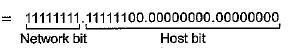6 bit are used for subnetting.
So number of possible subnets - 26 = 64.

QUESTION: 15

In a class A subnet, we know the IP address of one of the hosts and the mask as given below:
IP address = 25.34.12.56
Mask = 255.255.0.0

What is the first address (network address)?

Solution: